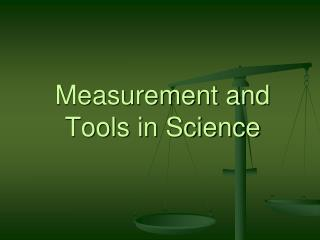DownloadDownload PresentationMeasurement and Tools in Science

# Measurement and Tools in Science

Télécharger la présentation## Measurement and Tools in Science

- - - - - - - - - - - - - - - - - - - - - - - - - - - E N D - - - - - - - - - - - - - - - - - - - - - - - - - - -
##### Presentation Transcript

1. Measurement and Tools in Science

2. Measurement • Measurement is a description of an object using numbers and units • Units in the US (for example: inches, gallons, and Fahrenheit) are different from units used by scientists • Scientists use the metric system also known as the International System of Units (SI for short) • The SI system is used by scientists for consistency

3. What are some units used in the SI System? • Degrees Celsius or Kelvin • Meters • Liters • Grams • Complete Part 2 on your worksheet now. • Answers: 1. C 2. B 3. D 4. A

4. Tools • In order for scientists to perform measurements we use tools. • What are some tools used by scientists for measurement? • Rulers • Balances • Graduated cylinders • Beakers • Thermometers • Droppers • Complete Part 3 on your worksheet now • Answers: 1. D 2. E 3. C 4. A 5. B 6. F

5. Each tool measures different values and uses different units for the measurement • Complete Parts 4 and 5 on your worksheet now • Answers Part 4: 1. A 2. B 3. C 4. C 5. B 6. D • Answers Part 5: 1. A 2. B 3.C 4. C 5. B 6. D